Publicité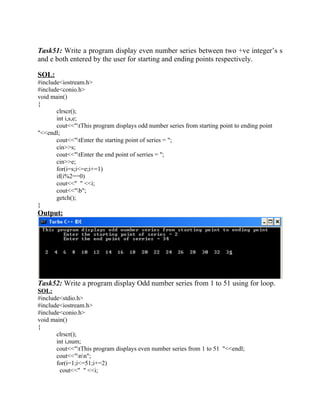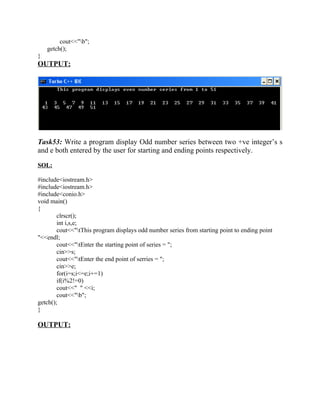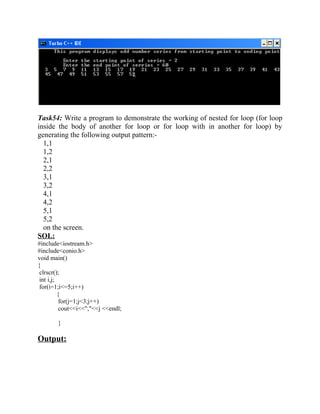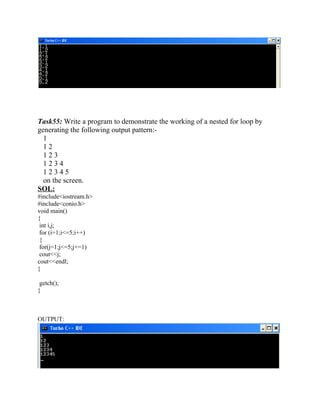Publicité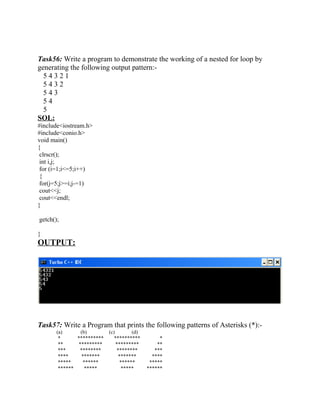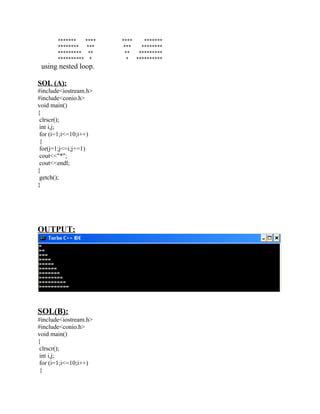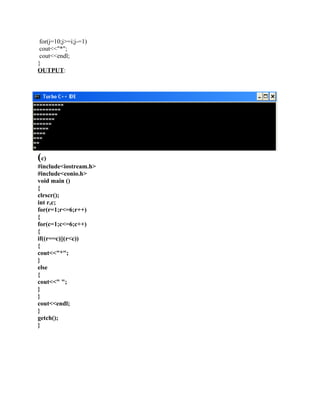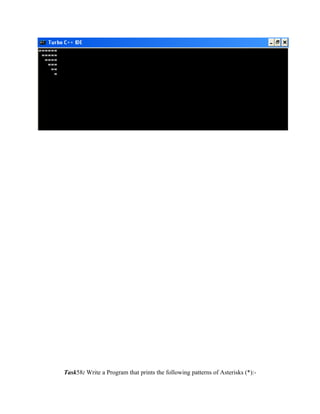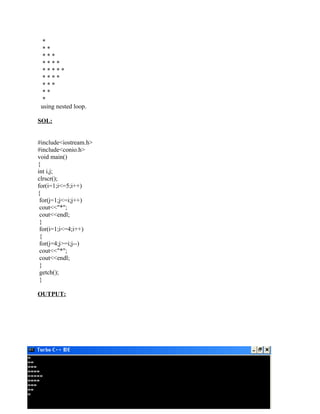Publicité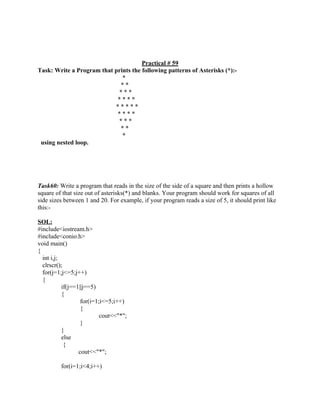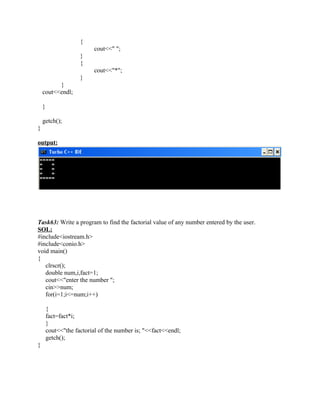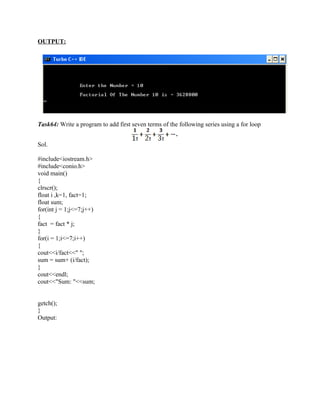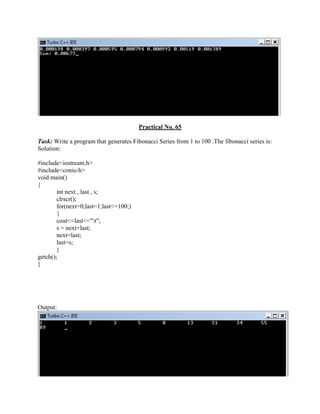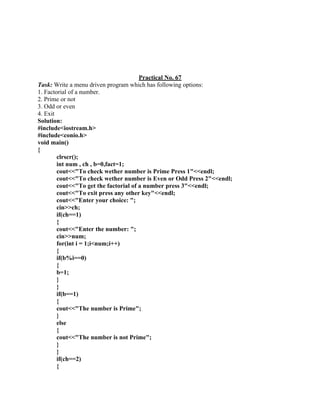Publicité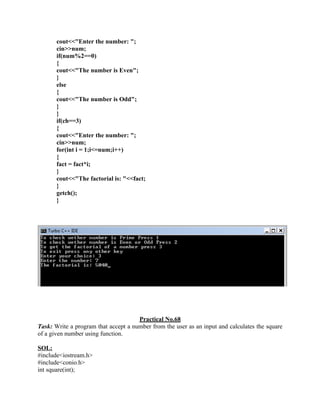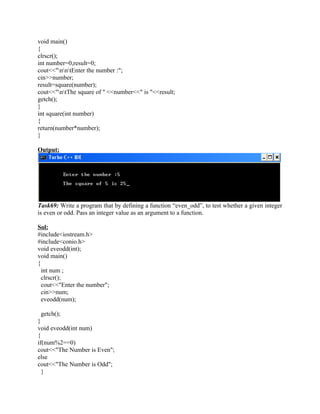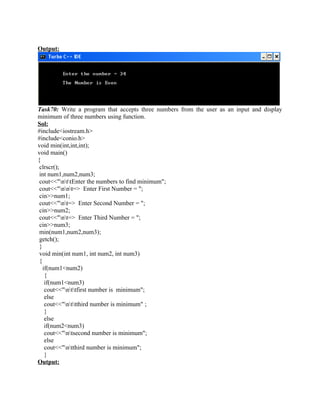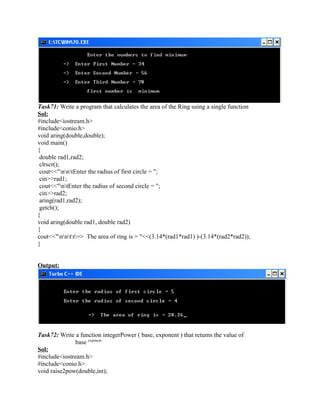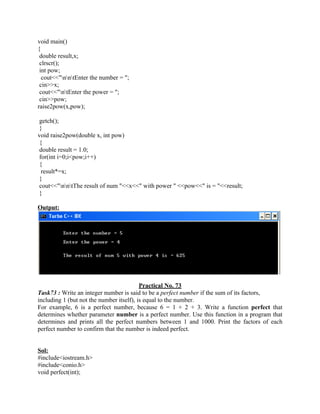Publicité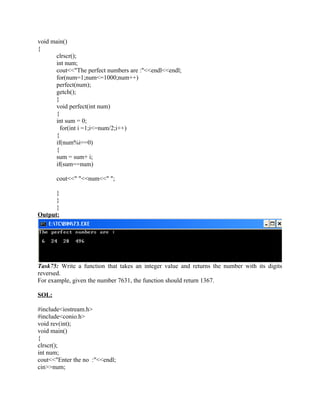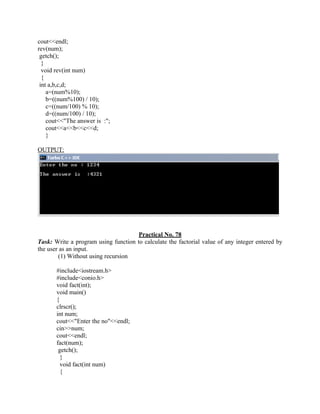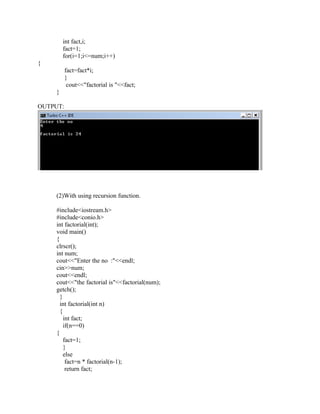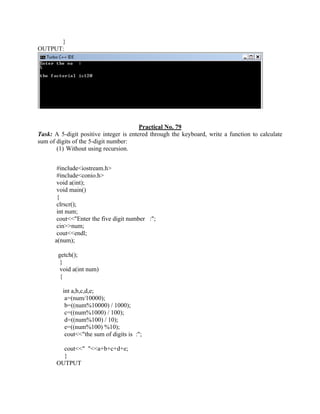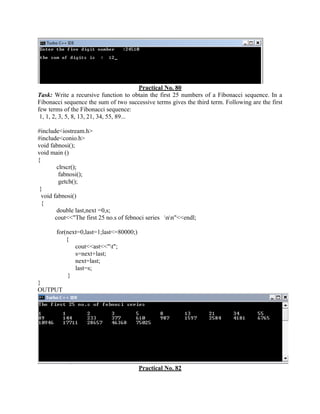Publicité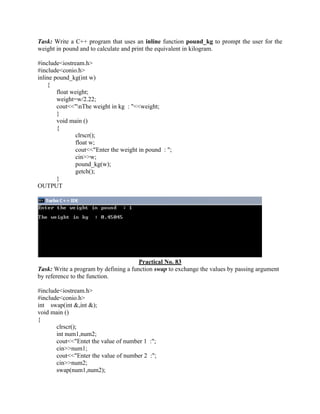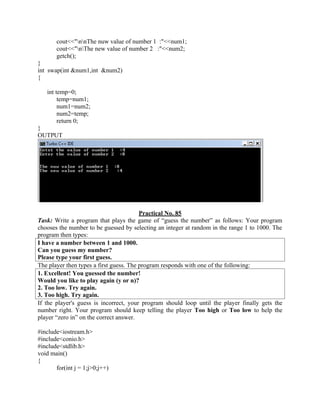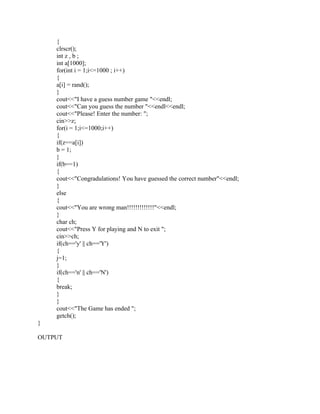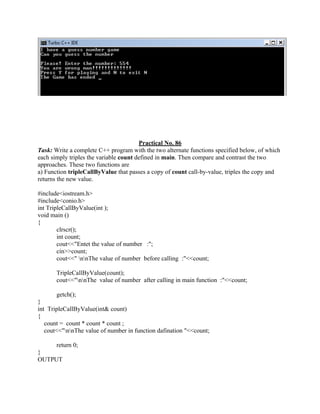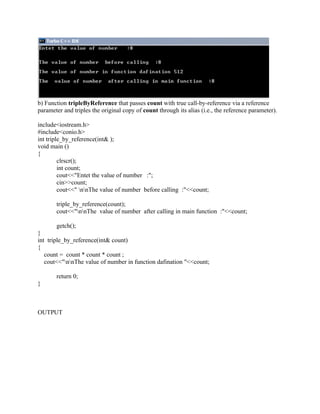Publicité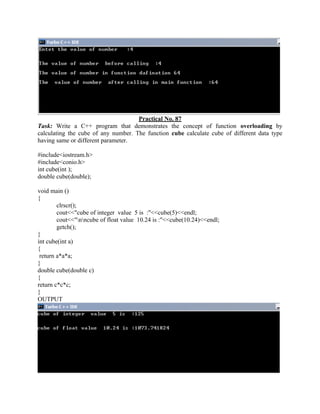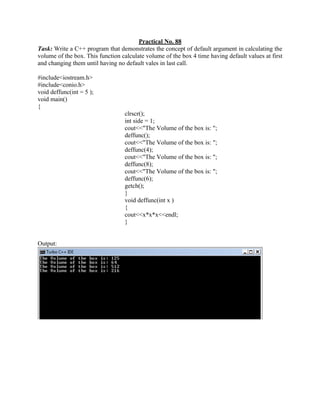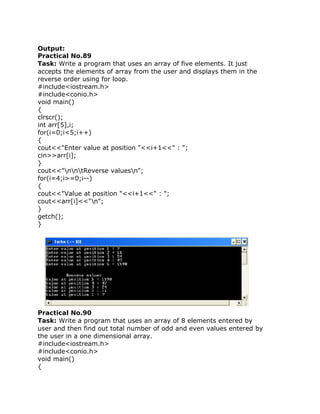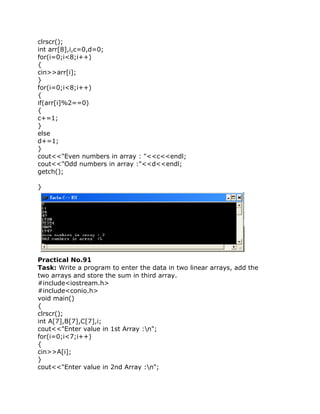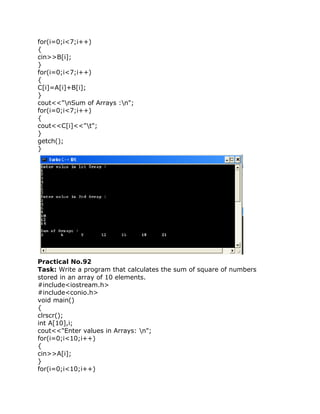Publicité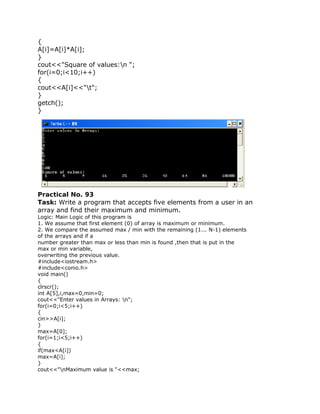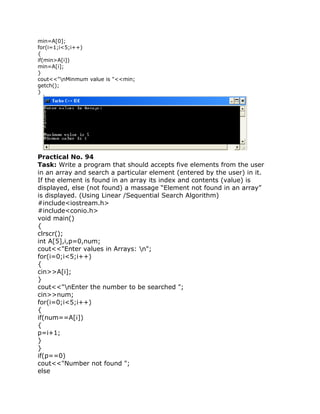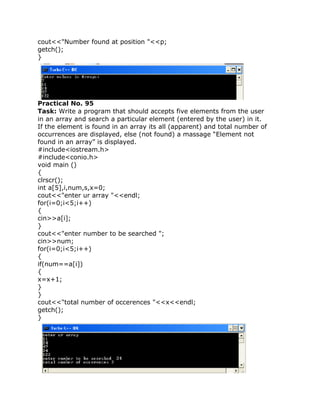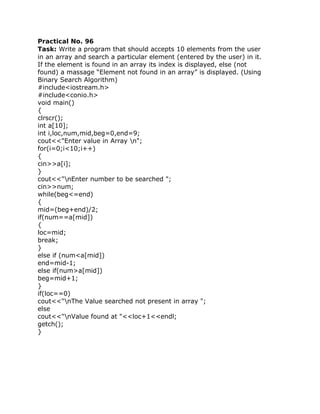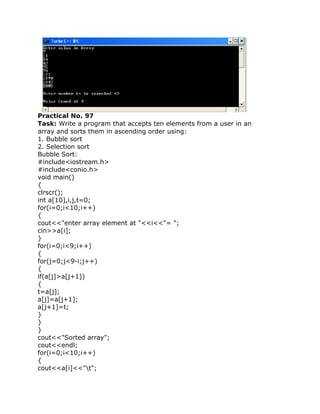Publicité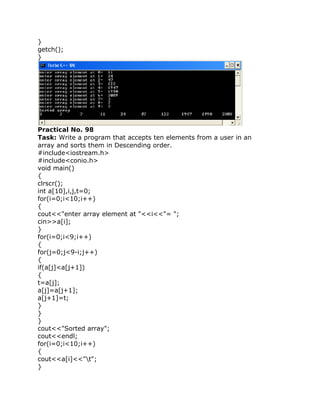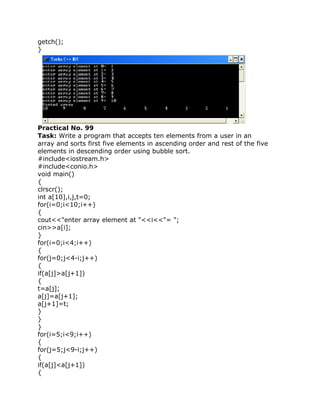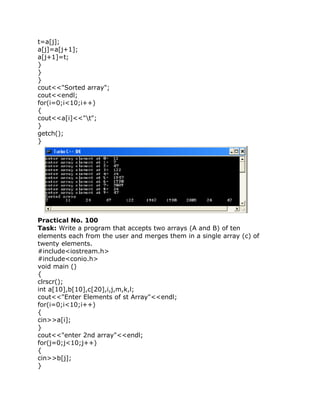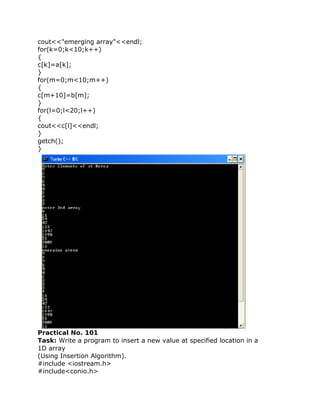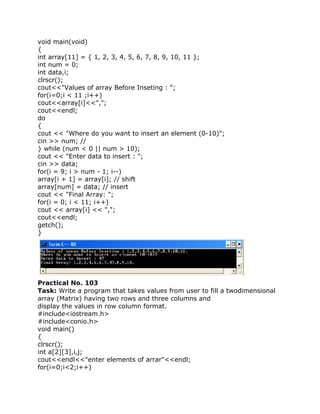Publicité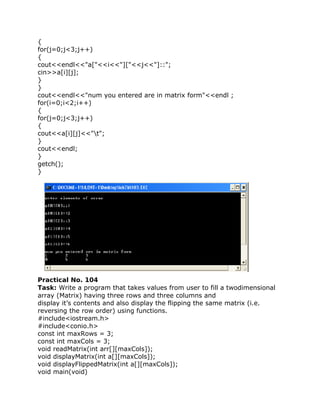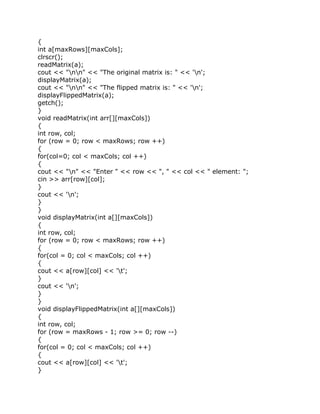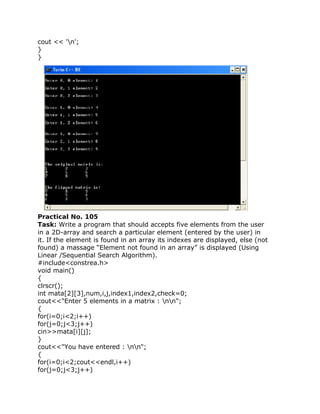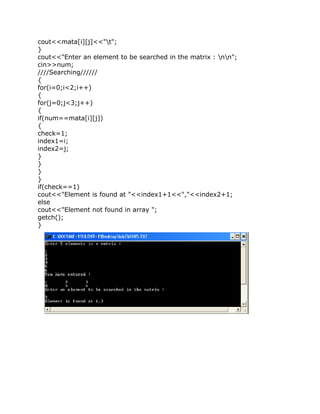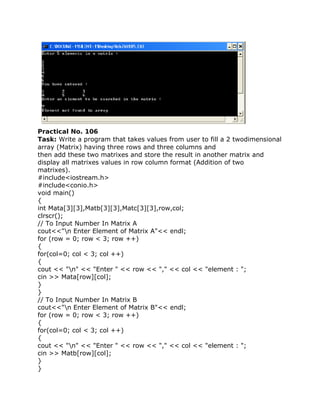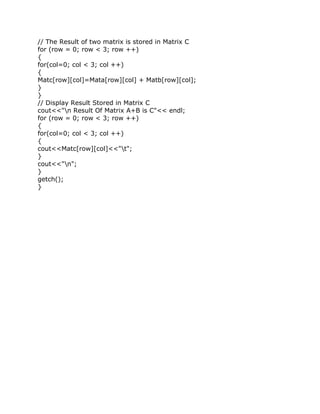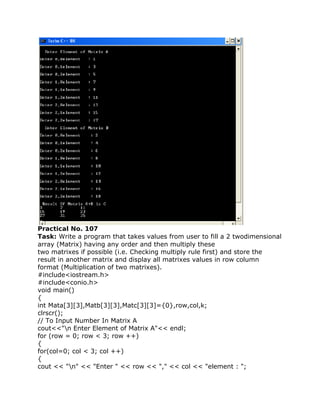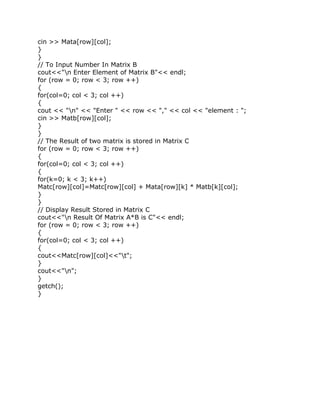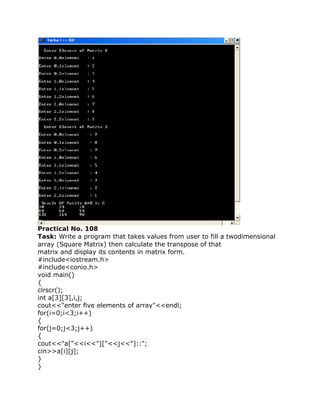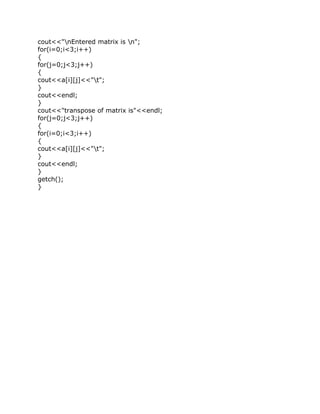Prochain SlideShare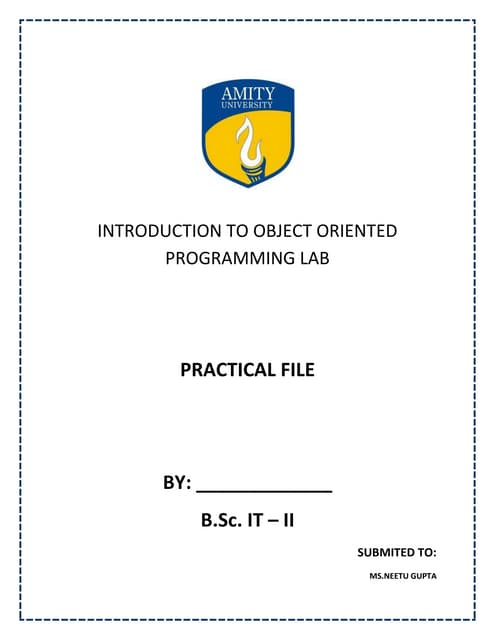C++ file
Chargement dans ... 3
1 sur 54
Publicité

### 54602399 c-examples-51-to-108-programe-ee01083101

1. Task51: Write a program display even number series between two +ve integer’s s and e both entered by the user for starting and ending points respectively. SOL: #include<iostream.h> #include<conio.h> void main() { clrscr(); int i,s,e; cout<<"tThis program displays odd number series from starting point to ending point "<<endl; cout<<"tEnter the starting point of series = "; cin>>s; cout<<"tEnter the end point of serries = "; cin>>e; for(i=s;i<=e;i+=1) if(i%2==0) cout<<" " <<i; cout<<"b"; getch(); } Output: Task52: Write a program display Odd number series from 1 to 51 using for loop. SOL: #include<stdio.h> #include<iostream.h> #include<conio.h> void main() { clrscr(); int i,num; cout<<"tThis program displays even number series from 1 to 51 "<<endl; cout<<"nn"; for(i=1;i<=51;i+=2) cout<<" " <<i;
2. cout<<"b"; getch(); } OUTPUT: Task53: Write a program display Odd number series between two +ve integer’s s and e both entered by the user for starting and ending points respectively. SOL: #include<iostream.h> #include<iostream.h> #include<conio.h> void main() { clrscr(); int i,s,e; cout<<"tThis program displays odd number series from starting point to ending point "<<endl; cout<<"tEnter the starting point of series = "; cin>>s; cout<<"tEnter the end point of serries = "; cin>>e; for(i=s;i<=e;i+=1) if(i%2!=0) cout<<" " <<i; cout<<"b"; getch(); } OUTPUT:
3. Task54: Write a program to demonstrate the working of nested for loop (for loop inside the body of another for loop or for loop with in another for loop) by generating the following output pattern:- 1,1 1,2 2,1 2,2 3,1 3,2 4,1 4,2 5,1 5,2 on the screen. SOL: #include<iostream.h> #include<conio.h> void main() { clrscr(); int i,j; for(i=1;i<=5;i++) { for(j=1;j<3;j++) cout<<i<<","<<j <<endl; } Output:
4. Task55: Write a program to demonstrate the working of a nested for loop by generating the following output pattern:- 1 12 123 1234 12345 on the screen. SOL: #include<iostream.h> #include<conio.h> void main() { int i,j; for (i=1;i<=5;i++) { for(j=1;j<=5;j+=1) cout<<j; cout<<endl; } getch(); } OUTPUT:
5. Task56: Write a program to demonstrate the working of a nested for loop by generating the following output pattern:- 54321 5432 543 54 5 SOL: #include<iostream.h> #include<conio.h> void main() { clrscr(); int i,j; for (i=1;i<=5;i++) { for(j=5;j>=i;j-=1) cout<<j; cout<<endl; } getch(); } OUTPUT: Task57: Write a Program that prints the following patterns of Asterisks (*):- (a) (b) (c) (d) * ********** ********** * ** ********* ********* ** *** ******** ******** *** **** ******* ******* **** ***** ****** ****** ***** ****** ***** ***** ******
6. ******* **** **** ******* ******** *** *** ******** ********* ** ** ********* ********** * * ********** using nested loop. SOL (A): #include<iostream.h> #include<conio.h> void main() { clrscr(); int i,j; for (i=1;i<=10;i++) { for(j=1;j<=i;j+=1) cout<<"*"; cout<<endl; } getch(); } OUTPUT: SOL(B): #include<iostream.h> #include<conio.h> void main() { clrscr(); int i,j; for (i=1;i<=10;i++) {
7. for(j=10;j>=i;j-=1) cout<<"*"; cout<<endl; } OUTPUT: (c) #include<iostream.h> #include<conio.h> void main () { clrscr(); int r,c; for(r=1;r<=6;r++) { for(c=1;c<=6;c++) { if((r==c)||(r<c)) { cout<<"*"; } else { cout<<" "; } } cout<<endl; } getch(); }
8. Task58: Write a Program that prints the following patterns of Asterisks (*):-
9. * ** *** **** ***** **** *** ** * using nested loop. SOL: #include<iostream.h> #include<conio.h> void main() { int i,j; clrscr(); for(i=1;i<=5;i++) { for(j=1;j<=i;j++) cout<<"*"; cout<<endl; } for(i=1;i<=4;i++) { for(j=4;j>=i;j--) cout<<"*"; cout<<endl; } getch(); } OUTPUT:
10. Practical # 59 Task: Write a Program that prints the following patterns of Asterisks (*):- * ** *** **** ***** **** *** ** * using nested loop. Task60: Write a program that reads in the size of the side of a square and then prints a hollow square of that size out of asterisks(*) and blanks. Your program should work for squares of all side sizes between 1 and 20. For example, if your program reads a size of 5, it should print like this:- SOL: #include<iostream.h> #include<conio.h> void main() { int i,j; clrscr(); for(j=1;j<=5;j++) { if(j==1||j==5) { for(i=1;i<=5;i++) { cout<<"*"; } } else { cout<<"*"; for(i=1;i<4;i++)
11. { cout<<" "; } { cout<<"*"; } } cout<<endl; } getch(); } output: Task63: Write a program to find the factorial value of any number entered by the user. SOL: #include<iostream.h> #include<conio.h> void main() { clrscr(); double num,i,fact=1; cout<<"enter the number "; cin>>num; for(i=1;i<=num;i++) { fact=fact*i; } cout<<"the factorial of the number is; "<<fact<<endl; getch(); }
12. OUTPUT: Task64: Write a program to add first seven terms of the following series using a for loop Sol. #include<iostream.h> #include<conio.h> void main() { clrscr(); float i ,k=1, fact=1; float sum; for(int j = 1;j<=7;j++) { fact = fact * j; } for(i = 1;i<=7;i++) { cout<<i/fact<<" "; sum = sum+ (i/fact); } cout<<endl; cout<<"Sum: "<<sum; getch(); } Output:
13. Practical No. 65 Task: Write a program that generates Fibonacci Series from 1 to 100 .The fibonacci series is: Solution: #include<iostream.h> #include<conio.h> void main() { int next , last , s; clrscr(); for(next=0,last=1;last<=100;) { cout<<last<<"t"; s = next+last; next=last; last=s; } getch(); } Output:
14. Practical No. 67 Task: Write a menu driven program which has following options: 1. Factorial of a number. 2. Prime or not 3. Odd or even 4. Exit Solution: #include<iostream.h> #include<conio.h> void main() { clrscr(); int num , ch , b=0,fact=1; cout<<"To check wether number is Prime Press 1"<<endl; cout<<"To check wether number is Even or Odd Press 2"<<endl; cout<<"To get the factorial of a number press 3"<<endl; cout<<"To exit press any other key"<<endl; cout<<"Enter your choice: "; cin>>ch; if(ch==1) { cout<<"Enter the number: "; cin>>num; for(int i = 1;i<num;i++) { if(b%i==0) { b=1; } } if(b==1) { cout<<"The number is Prime"; } else { cout<<"The number is not Prime"; } } if(ch==2) {
15. cout<<"Enter the number: "; cin>>num; if(num%2==0) { cout<<"The number is Even"; } else { cout<<"The number is Odd"; } } if(ch==3) { cout<<"Enter the number: "; cin>>num; for(int i = 1;i<=num;i++) { fact = fact*i; } cout<<"The factorial is: "<<fact; } getch(); } Practical No.68 Task: Write a program that accept a number from the user as an input and calculates the square of a given number using function. SOL: #include<iostream.h> #include<conio.h> int square(int);
16. void main() { clrscr(); int number=0,result=0; cout<<"nntEnter the number :"; cin>>number; result=square(number); cout<<"ntThe square of " <<number<<" is "<<result; getch(); } int square(int number) { return(number*number); } Output: Task69: Write a program that by defining a function “even_odd”, to test whether a given integer is even or odd. Pass an integer value as an argument to a function. Sol: #include<iostream.h> #include<conio.h> void eveodd(int); void main() { int num ; clrscr(); cout<<"Enter the number"; cin>>num; eveodd(num); getch(); } void eveodd(int num) { if(num%2==0) cout<<"The Number is Even"; else cout<<"The Number is Odd"; }
17. Output: Task70: Write a program that accepts three numbers from the user as an input and display minimum of three numbers using function. Sol: #include<iostream.h> #include<conio.h> void min(int,int,int); void main() { clrscr(); int num1,num2,num3; cout<<"nttEnter the numbers to find minimum"; cout<<"nnt=> Enter First Number = "; cin>>num1; cout<<"nt=> Enter Second Number = "; cin>>num2; cout<<"nt=> Enter Third Number = "; cin>>num3; min(num1,num2,num3); getch(); } void min(int num1, int num2, int num3) { if(num1<num2) { if(num1<num3) cout<<"nttfirst number is minimum"; else cout<<"nttthird number is minimum" ; } else if(num2<num3) cout<<"ntsecond number is minimum"; else cout<<"ntthird number is minimum"; } Output:
19. void main() { double result,x; clrscr(); int pow; cout<<"nntEnter the number = "; cin>>x; cout<<"ntEnter the power = "; cin>>pow; raise2pow(x,pow); getch(); } void raise2pow(double x, int pow) { double result = 1.0; for(int i=0;i<pow;i++) { result*=x; } cout<<"nntThe result of num "<<x<<" with power " <<pow<<" is = "<<result; } Output: Practical No. 73 Task73 : Write an integer number is said to be a perfect number if the sum of its factors, including 1 (but not the number itself), is equal to the number. For example, 6 is a perfect number, because 6 = 1 + 2 + 3. Write a function perfect that determines whether parameter number is a perfect number. Use this function in a program that determines and prints all the perfect numbers between 1 and 1000. Print the factors of each perfect number to confirm that the number is indeed perfect. Sol: #include<iostream.h> #include<conio.h> void perfect(int);
20. void main() { clrscr(); int num; cout<<"The perfect numbers are :"<<endl<<endl; for(num=1;num<=1000;num++) perfect(num); getch(); } void perfect(int num) { int sum = 0; for(int i =1;i<=num/2;i++) { if(num%i==0) { sum = sum+ i; if(sum==num) cout<<" "<<num<<" "; } } } Output: Task75: Write a function that takes an integer value and returns the number with its digits reversed. For example, given the number 7631, the function should return 1367. SOL: #include<iostream.h> #include<conio.h> void rev(int); void main() { clrscr(); int num; cout<<"Enter the no :"<<endl; cin>>num;
21. cout<<endl; rev(num); getch(); } void rev(int num) { int a,b,c,d; a=(num%10); b=((num%100) / 10); c=((num/100) % 10); d=((num/100) / 10); cout<<"The answer is :"; cout<<a<<b<<c<<d; } OUTPUT: Practical No. 78 Task: Write a program using function to calculate the factorial value of any integer entered by the user as an input. (1) Without using recursion #include<iostream.h> #include<conio.h> void fact(int); void main() { clrscr(); int num; cout<<"Enter the no"<<endl; cin>>num; cout<<endl; fact(num); getch(); } void fact(int num) {
22. int fact,i; fact=1; for(i=1;i<=num;i++) { fact=fact*i; } cout<<"factorial is "<<fact; } OUTPUT: (2)With using recursion function. #include<iostream.h> #include<conio.h> int factorial(int); void main() { clrscr(); int num; cout<<"Enter the no :"<<endl; cin>>num; cout<<endl; cout<<"the factorial is"<<factorial(num); getch(); } int factorial(int n) { int fact; if(n==0) { fact=1; } else fact=n * factorial(n-1); return fact;
23. } OUTPUT: Practical No. 79 Task: A 5-digit positive integer is entered through the keyboard, write a function to calculate sum of digits of the 5-digit number: (1) Without using recursion. #include<iostream.h> #include<conio.h> void a(int); void main() { clrscr(); int num; cout<<"Enter the five digit number :"; cin>>num; cout<<endl; a(num); getch(); } void a(int num) { int a,b,c,d,e; a=(num/10000); b=((num%10000) / 1000); c=((num%1000) / 100); d=((num%100) / 10); e=((num%100) %10); cout<<"the sum of digits is :"; cout<<" "<<a+b+c+d+e; } OUTPUT
24. Practical No. 80 Task: Write a recursive function to obtain the first 25 numbers of a Fibonacci sequence. In a Fibonacci sequence the sum of two successive terms gives the third term. Following are the first few terms of the Fibonacci sequence: 1, 1, 2, 3, 5, 8, 13, 21, 34, 55, 89... #include<iostream.h> #include<conio.h> void fabnosi(); void main () { clrscr(); fabnosi(); getch(); } void fabnosi() { double last,next =0,s; cout<<"The first 25 no.s of febnoci series nn"<<endl; for(next=0,last=1;last<=80000;) { cout<<ast<<"t"; s=next+last; next=last; last=s; } } OUTPUT Practical No. 82
25. Task: Write a C++ program that uses an inline function pound_kg to prompt the user for the weight in pound and to calculate and print the equivalent in kilogram. #include<iostream.h> #include<conio.h> inline pound_kg(int w) { float weight; weight=w/2.22; cout<<"nThe weight in kg : "<<weight; } void main () { clrscr(); float w; cout<<"Enter the weight in pound : "; cin>>w; pound_kg(w); getch(); } OUTPUT Practical No. 83 Task: Write a program by defining a function swap to exchange the values by passing argument by reference to the function. #include<iostream.h> #include<conio.h> int swap(int &,int &); void main () { clrscr(); int num1,num2; cout<<"Entet the value of number 1 :"; cin>>num1; cout<<"Enter the value of number 2 :"; cin>>num2; swap(num1,num2);
26. cout<<"nnThe nuw value of number 1 :"<<num1; cout<<"nThe new value of number 2 :"<<num2; getch(); } int swap(int &num1,int &num2) { int temp=0; temp=num1; num1=num2; num2=temp; return 0; } OUTPUT Practical No. 85 Task: Write a program that plays the game of “guess the number” as follows: Your program chooses the number to be guessed by selecting an integer at random in the range 1 to 1000. The program then types: I have a number between 1 and 1000. Can you guess my number? Please type your first guess. The player then types a first guess. The program responds with one of the following: 1. Excellent! You guessed the number! Would you like to play again (y or n)? 2. Too low. Try again. 3. Too high. Try again. If the player's guess is incorrect, your program should loop until the player finally gets the number right. Your program should keep telling the player Too high or Too low to help the player “zero in” on the correct answer. #include<iostream.h> #include<conio.h> #include<stdlib.h> void main() { for(int j = 1;j>0;j++)
27. { clrscr(); int z , b ; int a; for(int i = 1;i<=1000 ; i++) { a[i] = rand(); } cout<<"I have a guess number game "<<endl; cout<<"Can you guess the number "<<endl<<endl; cout<<"Please! Enter the number: "; cin>>z; for(i = 1;i<=1000;i++) { if(z==a[i]) b = 1; } if(b==1) { cout<<"Congradulations! You have guessed the correct number"<<endl; } else { cout<<"You are wrong man!!!!!!!!!!!!!"<<endl; } char ch; cout<<"Press Y for playing and N to exit "; cin>>ch; if(ch=='y' || ch=='Y') { j=1; } if(ch=='n' || ch=='N') { break; } } cout<<"The Game has ended "; getch(); } OUTPUT
28. Practical No. 86 Task: Write a complete C++ program with the two alternate functions specified below, of which each simply triples the variable count defined in main. Then compare and contrast the two approaches. These two functions are a) Function tripleCallByValue that passes a copy of count call-by-value, triples the copy and returns the new value. #include<iostream.h> #include<conio.h> int TripleCallByValue(int ); void main () { clrscr(); int count; cout<<"Entet the value of number :"; cin>>count; cout<<" nnThe value of number before calling :"<<count; TripleCallByValue(count); cout<<"nnThe value of number after calling in main function :"<<count; getch(); } int TripleCallByValue(int& count) { count = count * count * count ; cout<<"nnThe value of number in function dafination "<<count; return 0; } OUTPUT
29. b) Function tripleByReference that passes count with true call-by-reference via a reference parameter and triples the original copy of count through its alias (i.e., the reference parameter). include<iostream.h> #include<conio.h> int triple_by_reference(int& ); void main () { clrscr(); int count; cout<<"Entet the value of number :"; cin>>count; cout<<" nnThe value of number before calling :"<<count; triple_by_reference(count); cout<<"nnThe value of number after calling in main function :"<<count; getch(); } int triple_by_reference(int& count) { count = count * count * count ; cout<<"nnThe value of number in function dafination "<<count; return 0; } OUTPUT
30. Practical No. 87 Task: Write a C++ program that demonstrates the concept of function overloading by calculating the cube of any number. The function cube calculate cube of different data type having same or different parameter. #include<iostream.h> #include<conio.h> int cube(int ); double cube(double); void main () { clrscr(); cout<<"cube of integer value 5 is :"<<cube(5)<<endl; cout<<"nncube of float value 10.24 is :"<<cube(10.24)<<endl; getch(); } int cube(int a) { return a*a*a; } double cube(double c) { return c*c*c; } OUTPUT
31. Practical No. 88 Task: Write a C++ program that demonstrates the concept of default argument in calculating the volume of the box. This function calculate volume of the box 4 time having default values at first and changing them until having no default vales in last call. #include<iostream.h> #include<conio.h> void deffunc(int = 5 ); void main() { clrscr(); int side = 1; cout<<"The Volume of the box is: "; deffunc(); cout<<"The Volume of the box is: "; deffunc(4); cout<<"The Volume of the box is: "; deffunc(8); cout<<"The Volume of the box is: "; deffunc(6); getch(); } void deffunc(int x ) { cout<<x*x*x<<endl; } Output:
32. Output: Practical No.89 Task: Write a program that uses an array of five elements. It just accepts the elements of array from the user and displays them in the reverse order using for loop. #include<iostream.h> #include<conio.h> void main() { clrscr(); int arr,i; for(i=0;i<5;i++) { cout<<"Enter value at position "<<i+1<<" : "; cin>>arr[i]; } cout<<"nntReverse valuesn"; for(i=4;i>=0;i--) { cout<<"Value at position "<<i+1<<" : "; cout<<arr[i]<<"n"; } getch(); } Practical No.90 Task: Write a program that uses an array of 8 elements entered by user and then find out total number of odd and even values entered by the user in a one dimensional array. #include<iostream.h> #include<conio.h> void main() {
33. clrscr(); int arr,i,c=0,d=0; for(i=0;i<8;i++) { cin>>arr[i]; } for(i=0;i<8;i++) { if(arr[i]%2==0) { c+=1; } else d+=1; } cout<<"Even numbers in array : "<<c<<endl; cout<<"Odd numbers in array :"<<d<<endl; getch(); } Practical No.91 Task: Write a program to enter the data in two linear arrays, add the two arrays and store the sum in third array. #include<iostream.h> #include<conio.h> void main() { clrscr(); int A,B,C,i; cout<<"Enter value in 1st Array :n"; for(i=0;i<7;i++) { cin>>A[i]; } cout<<"Enter value in 2nd Array :n";
34. for(i=0;i<7;i++) { cin>>B[i]; } for(i=0;i<7;i++) { C[i]=A[i]+B[i]; } cout<<"nSum of Arrays :n"; for(i=0;i<7;i++) { cout<<C[i]<<"t"; } getch(); } Practical No.92 Task: Write a program that calculates the sum of square of numbers stored in an array of 10 elements. #include<iostream.h> #include<conio.h> void main() { clrscr(); int A,i; cout<<"Enter values in Arrays: n"; for(i=0;i<10;i++) { cin>>A[i]; } for(i=0;i<10;i++)
35. { A[i]=A[i]*A[i]; } cout<<"Square of values:n "; for(i=0;i<10;i++) { cout<<A[i]<<"t"; } getch(); } Practical No. 93 Task: Write a program that accepts five elements from a user in an array and find their maximum and minimum. Logic: Main Logic of this program is 1. We assume that first element (0) of array is maximum or minimum. 2. We compare the assumed max / min with the remaining (1... N-1) elements of the arrays and if a number greater than max or less than min is found ,then that is put in the max or min variable, overwriting the previous value. #include<iostream.h> #include<conio.h> void main() { clrscr(); int A,i,max=0,min=0; cout<<"Enter values in Arrays: n"; for(i=0;i<5;i++) { cin>>A[i]; } max=A; for(i=1;i<5;i++) { if(max<A[i]) max=A[i]; } cout<<"nMaximum value is "<<max;
36. min=A; for(i=1;i<5;i++) { if(min>A[i]) min=A[i]; } cout<<"nMinmum value is "<<min; getch(); } Practical No. 94 Task: Write a program that should accepts five elements from the user in an array and search a particular element (entered by the user) in it. If the element is found in an array its index and contents (value) is displayed, else (not found) a massage “Element not found in an array” is displayed. (Using Linear /Sequential Search Algorithm) #include<iostream.h> #include<conio.h> void main() { clrscr(); int A,i,p=0,num; cout<<"Enter values in Arrays: n"; for(i=0;i<5;i++) { cin>>A[i]; } cout<<"nEnter the number to be searched "; cin>>num; for(i=0;i<5;i++) { if(num==A[i]) { p=i+1; } } if(p==0) cout<<"Number not found "; else
37. cout<<"Number found at position "<<p; getch(); } Practical No. 95 Task: Write a program that should accepts five elements from the user in an array and search a particular element (entered by the user) in it. If the element is found in an array its all (apparent) and total number of occurrences are displayed, else (not found) a massage “Element not found in an array” is displayed. #include<iostream.h> #include<conio.h> void main () { clrscr(); int a,i,num,s,x=0; cout<<"enter ur array "<<endl; for(i=0;i<5;i++) { cin>>a[i]; } cout<<"enter number to be searched "; cin>>num; for(i=0;i<5;i++) { if(num==a[i]) { x=x+1; } } cout<<"total number of occerences "<<x<<endl; getch(); }
38. Practical No. 96 Task: Write a program that should accepts 10 elements from the user in an array and search a particular element (entered by the user) in it. If the element is found in an array its index is displayed, else (not found) a massage “Element not found in an array” is displayed. (Using Binary Search Algorithm) #include<iostream.h> #include<conio.h> void main() { clrscr(); int a; int i,loc,num,mid,beg=0,end=9; cout<<"Enter value in Array n"; for(i=0;i<10;i++) { cin>>a[i]; } cout<<"nEnter number to be searched "; cin>>num; while(beg<=end) { mid=(beg+end)/2; if(num==a[mid]) { loc=mid; break; } else if (num<a[mid]) end=mid-1; else if(num>a[mid]) beg=mid+1; } if(loc==0) cout<<"nThe Value searched not present in array "; else cout<<"nValue found at "<<loc+1<<endl; getch(); }
39. Practical No. 97 Task: Write a program that accepts ten elements from a user in an array and sorts them in ascending order using: 1. Bubble sort 2. Selection sort Bubble Sort: #include<iostream.h> #include<conio.h> void main() { clrscr(); int a,i,j,t=0; for(i=0;i<10;i++) { cout<<"enter array element at "<<i<<"= "; cin>>a[i]; } for(i=0;i<9;i++) { for(j=0;j<9-i;j++) { if(a[j]>a[j+1]) { t=a[j]; a[j]=a[j+1]; a[j+1]=t; } } } cout<<"Sorted array"; cout<<endl; for(i=0;i<10;i++) { cout<<a[i]<<"t";
40. } getch(); } Practical No. 98 Task: Write a program that accepts ten elements from a user in an array and sorts them in Descending order. #include<iostream.h> #include<conio.h> void main() { clrscr(); int a,i,j,t=0; for(i=0;i<10;i++) { cout<<"enter array element at "<<i<<"= "; cin>>a[i]; } for(i=0;i<9;i++) { for(j=0;j<9-i;j++) { if(a[j]<a[j+1]) { t=a[j]; a[j]=a[j+1]; a[j+1]=t; } } } cout<<"Sorted array"; cout<<endl; for(i=0;i<10;i++) { cout<<a[i]<<"t"; }
41. getch(); } Practical No. 99 Task: Write a program that accepts ten elements from a user in an array and sorts first five elements in ascending order and rest of the five elements in descending order using bubble sort. #include<iostream.h> #include<conio.h> void main() { clrscr(); int a,i,j,t=0; for(i=0;i<10;i++) { cout<<"enter array element at "<<i<<"= "; cin>>a[i]; } for(i=0;i<4;i++) { for(j=0;j<4-i;j++) { if(a[j]>a[j+1]) { t=a[j]; a[j]=a[j+1]; a[j+1]=t; } } } for(i=5;i<9;i++) { for(j=5;j<9-i;j++) { if(a[j]<a[j+1]) {
42. t=a[j]; a[j]=a[j+1]; a[j+1]=t; } } } cout<<"Sorted array"; cout<<endl; for(i=0;i<10;i++) { cout<<a[i]<<"t"; } getch(); } Practical No. 100 Task: Write a program that accepts two arrays (A and B) of ten elements each from the user and merges them in a single array (c) of twenty elements. #include<iostream.h> #include<conio.h> void main () { clrscr(); int a,b,c,i,j,m,k,l; cout<<"Enter Elements of st Array"<<endl; for(i=0;i<10;i++) { cin>>a[i]; } cout<<"enter 2nd array"<<endl; for(j=0;j<10;j++) { cin>>b[j]; }
43. cout<<"emerging array"<<endl; for(k=0;k<10;k++) { c[k]=a[k]; } for(m=0;m<10;m++) { c[m+10]=b[m]; } for(l=0;l<20;l++) { cout<<c[l]<<endl; } getch(); } Practical No. 101 Task: Write a program to insert a new value at specified location in a 1D array (Using Insertion Algorithm). #include <iostream.h> #include<conio.h>
44. void main(void) { int array = { 1, 2, 3, 4, 5, 6, 7, 8, 9, 10, 11 }; int num = 0; int data,i; clrscr(); cout<<"Values of array Before Inseting : "; for(i=0;i < 11 ;i++) cout<<array[i]<<","; cout<<endl; do { cout << "Where do you want to insert an element (0-10)"; cin >> num; // } while (num < 0 || num > 10); cout << "Enter data to insert : "; cin >> data; for(i = 9; i > num - 1; i--) array[i + 1] = array[i]; // shift array[num] = data; // insert cout << "Final Array: "; for(i = 0; i < 11; i++) cout << array[i] << ","; cout<<endl; getch(); } Practical No. 103 Task: Write a program that takes values from user to fill a twodimensional array (Matrix) having two rows and three columns and display the values in row column format. #include<iostream.h> #include<conio.h> void main() { clrscr(); int a,i,j; cout<<endl<<"enter elements of arrar"<<endl; for(i=0;i<2;i++)
45. { for(j=0;j<3;j++) { cout<<endl<<"a["<<i<<"]["<<j<<"]::"; cin>>a[i][j]; } } cout<<endl<<"num you entered are in matrix form"<<endl ; for(i=0;i<2;i++) { for(j=0;j<3;j++) { cout<<a[i][j]<<"t"; } cout<<endl; } getch(); } Practical No. 104 Task: Write a program that takes values from user to fill a twodimensional array (Matrix) having three rows and three columns and display it’s contents and also display the flipping the same matrix (i.e. reversing the row order) using functions. #include<iostream.h> #include<conio.h> const int maxRows = 3; const int maxCols = 3; void readMatrix(int arr[][maxCols]); void displayMatrix(int a[][maxCols]); void displayFlippedMatrix(int a[][maxCols]); void main(void)
46. { int a[maxRows][maxCols]; clrscr(); readMatrix(a); cout << "nn" << "The original matrix is: " << 'n'; displayMatrix(a); cout << "nn" << "The flipped matrix is: " << 'n'; displayFlippedMatrix(a); getch(); } void readMatrix(int arr[][maxCols]) { int row, col; for (row = 0; row < maxRows; row ++) { for(col=0; col < maxCols; col ++) { cout << "n" << "Enter " << row << ", " << col << " element: "; cin >> arr[row][col]; } cout << 'n'; } } void displayMatrix(int a[][maxCols]) { int row, col; for (row = 0; row < maxRows; row ++) { for(col = 0; col < maxCols; col ++) { cout << a[row][col] << 't'; } cout << 'n'; } } void displayFlippedMatrix(int a[][maxCols]) { int row, col; for (row = maxRows - 1; row >= 0; row --) { for(col = 0; col < maxCols; col ++) { cout << a[row][col] << 't'; }
47. cout << 'n'; } } Practical No. 105 Task: Write a program that should accepts five elements from the user in a 2D-array and search a particular element (entered by the user) in it. If the element is found in an array its indexes are displayed, else (not found) a massage “Element not found in an array” is displayed (Using Linear /Sequential Search Algorithm). #include<constrea.h> void main() { clrscr(); int mata,num,i,j,index1,index2,check=0; cout<<"Enter 5 elements in a matrix : nn"; { for(i=0;i<2;i++) for(j=0;j<3;j++) cin>>mata[i][j]; } cout<<"You have entered : nn"; { for(i=0;i<2;cout<<endl,i++) for(j=0;j<3;j++)
48. cout<<mata[i][j]<<"t"; } cout<<"Enter an element to be searched in the matrix : nn"; cin>>num; ////Searching////// { for(i=0;i<2;i++) { for(j=0;j<3;j++) { if(num==mata[i][j]) { check=1; index1=i; index2=j; } } } } if(check==1) cout<<"Element is found at "<<index1+1<<","<<index2+1; else cout<<"Element not found in array "; getch(); }
49. Practical No. 106 Task: Write a program that takes values from user to fill a 2 twodimensional array (Matrix) having three rows and three columns and then add these two matrixes and store the result in another matrix and display all matrixes values in row column format (Addition of two matrixes). #include<iostream.h> #include<conio.h> void main() { int Mata,Matb,Matc,row,col; clrscr(); // To Input Number In Matrix A cout<<"n Enter Element of Matrix A"<< endl; for (row = 0; row < 3; row ++) { for(col=0; col < 3; col ++) { cout << "n" << "Enter " << row << "," << col << "element : "; cin >> Mata[row][col]; } } // To Input Number In Matrix B cout<<"n Enter Element of Matrix B"<< endl; for (row = 0; row < 3; row ++) { for(col=0; col < 3; col ++) { cout << "n" << "Enter " << row << "," << col << "element : "; cin >> Matb[row][col]; } }
50. // The Result of two matrix is stored in Matrix C for (row = 0; row < 3; row ++) { for(col=0; col < 3; col ++) { Matc[row][col]=Mata[row][col] + Matb[row][col]; } } // Display Result Stored in Matrix C cout<<"n Result Of Matrix A+B is C"<< endl; for (row = 0; row < 3; row ++) { for(col=0; col < 3; col ++) { cout<<Matc[row][col]<<"t"; } cout<<"n"; } getch(); }
51. Practical No. 107 Task: Write a program that takes values from user to fill a 2 twodimensional array (Matrix) having any order and then multiply these two matrixes if possible (i.e. Checking multiply rule first) and store the result in another matrix and display all matrixes values in row column format (Multiplication of two matrixes). #include<iostream.h> #include<conio.h> void main() { int Mata,Matb,Matc={0},row,col,k; clrscr(); // To Input Number In Matrix A cout<<"n Enter Element of Matrix A"<< endl; for (row = 0; row < 3; row ++) { for(col=0; col < 3; col ++) { cout << "n" << "Enter " << row << "," << col << "element : ";
52. cin >> Mata[row][col]; } } // To Input Number In Matrix B cout<<"n Enter Element of Matrix B"<< endl; for (row = 0; row < 3; row ++) { for(col=0; col < 3; col ++) { cout << "n" << "Enter " << row << "," << col << "element : "; cin >> Matb[row][col]; } } // The Result of two matrix is stored in Matrix C for (row = 0; row < 3; row ++) { for(col=0; col < 3; col ++) { for(k=0; k < 3; k++) Matc[row][col]=Matc[row][col] + Mata[row][k] * Matb[k][col]; } } // Display Result Stored in Matrix C cout<<"n Result Of Matrix A*B is C"<< endl; for (row = 0; row < 3; row ++) { for(col=0; col < 3; col ++) { cout<<Matc[row][col]<<"t"; } cout<<"n"; } getch(); }
53. Practical No. 108 Task: Write a program that takes values from user to fill a twodimensional array (Square Matrix) then calculate the transpose of that matrix and display its contents in matrix form. #include<iostream.h> #include<conio.h> void main() { clrscr(); int a,i,j; cout<<"enter five elements of array"<<endl; for(i=0;i<3;i++) { for(j=0;j<3;j++) { cout<<"a["<<i<<"]["<<j<<"]::"; cin>>a[i][j]; } }
54. cout<<"nEntered matrix is n"; for(i=0;i<3;i++) { for(j=0;j<3;j++) { cout<<a[i][j]<<"t"; } cout<<endl; } cout<<"transpose of matrix is"<<endl; for(j=0;j<3;j++) { for(i=0;i<3;i++) { cout<<a[i][j]<<"t"; } cout<<endl; } getch(); }
Publicité